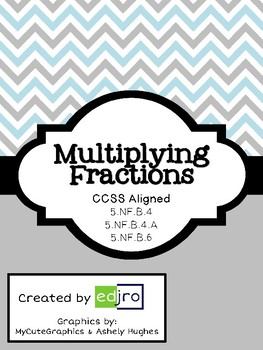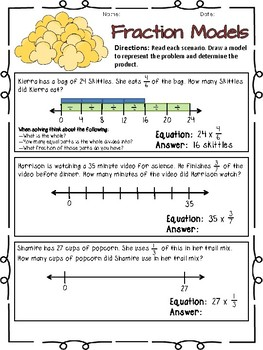Multiplying Fractions4th - 7th
Subjects
Standards
Resource Type
Formats Included
• PDF
Pages
15 pages

Description

The TEN printables included in this product are aligned to meet fifth grade standards, but can be used to enrich fourth graders or reteach sixth graders.

In the product students practice multiplying fractions by a whole number using a visual model and a numberline. There are three two-sided practice pages which can be used in small group, independent practice, homework, or as an assessment.

Also, the product includes multiplying two fractions using an area model or other strategy. The seven printable activities vary in difficulty. A teacher could use the different activities for differentiated groups. These printables can also be used to increase student confidence with the skill of multiplying fractions. Again these activities can be used in small group, independent practice, homework, or as an assessment.
Total Pages
15 pages
Not Included
Teaching Duration
N/A
Report this Resource to TpT
Reported resources will be reviewed by our team. Report this resource to let us know if this resource violates TpT’s content guidelines.

Standards

to see state-specific standards (only available in the US).
Solve real world problems involving multiplication of fractions and mixed numbers, e.g., by using visual fraction models or equations to represent the problem.
Interpret the product (𝘢/𝘣) × 𝘲 as a parts of a partition of 𝘲 into 𝘣 equal parts; equivalently, as the result of a sequence of operations 𝘢 × 𝘲 ÷ 𝘣. For example, use a visual fraction model to show (2/3) × 4 = 8/3, and create a story context for this equation. Do the same with (2/3) × (4/5) = 8/15. (In general, (𝘢/𝘣) × (𝘤/𝘥) = 𝘢𝘤/𝘣𝘥.)
Apply and extend previous understandings of multiplication to multiply a fraction or whole number by a fraction.